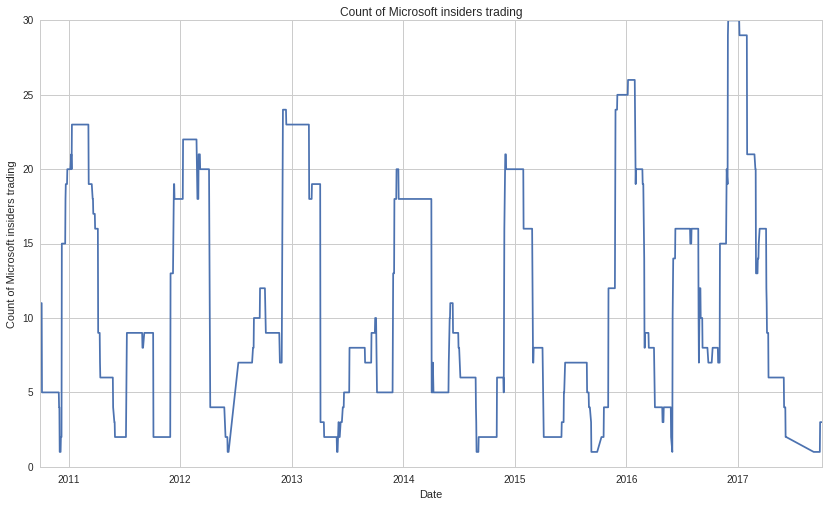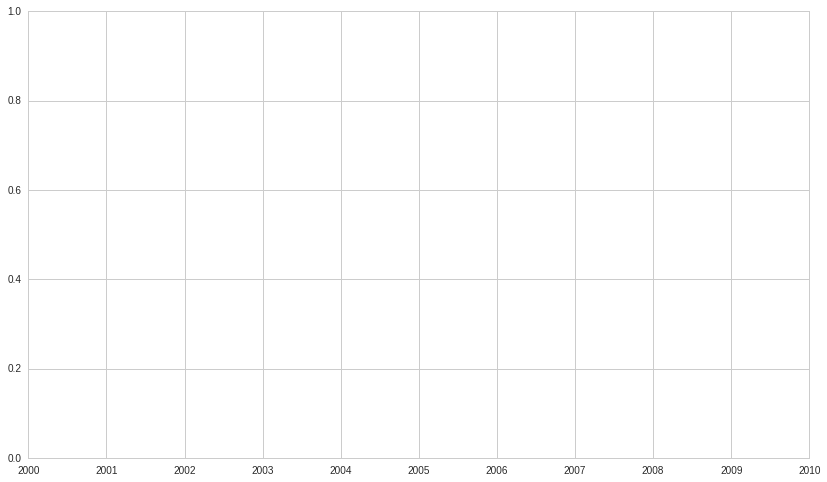Notebook
In :
import quantopian.optimize as opt
from quantopian.pipeline import Pipeline
from quantopian.pipeline.data.builtin import USEquityPricing
from quantopian.pipeline.domain import US_EQUITIES
from quantopian.research import run_pipeline

# Form 3 transactions
# Form 4 and Form 5 transactions

import pandas as pd
import numpy as np
import matplotlib.pyplot as plt
import seaborn as sns

In :
qtu = QTradableStocksUS()
# DataSetFamily into DataSets. Here, insider_txns_form3_90d is a DataSet
# containing insider transaction data for Form 3 over the past 90 calendar
# days, and insider_txns_form4and5_90d is a DataSet containing insider
# transaction data for Forms 4 and 5 over the past 90 calendar days. We only
# include non-derivative ownership (derivative_holdings is False).
# From each DataSet, extract the number of unique buyers and unique sellers.
# We do not need to include unique sellers using Form 3, because Form 3 is
# an initial ownership filing, and so there are no sellers using Form 3.
unique_filers_form3_90d = insider_txns_form3_90d.num_unique_filers.latest
unique_sellers_form4and5_90d = insider_txns_form4and5_90d.num_unique_sellers.latest
# Sum the unique buyers from each form together.
unique_sellers_90d = unique_sellers_form4and5_90d
# Compute the fractions of insiders buying and selling.
frac_insiders_selling_90d = unique_sellers_90d / (unique_buyers_90d + unique_sellers_90d)

# compute factor as buying-selling rank zscores

screen = qtu & ~alpha_factor.isnull() & alpha_factor.isfinite()

alpha_factor, screen

# Winsorize to remove extreme outliers
alpha_winsorized = alpha_factor.winsorize(min_percentile=0.02,
max_percentile=0.98,

# Zscore and rank to get long and short (positive and negative) alphas to use as weights
alpha_rank = alpha_winsorized.rank().zscore()

pipe = Pipeline(columns={'alpha_factor': alpha_rank},
screen=screen, domain=US_EQUITIES)

results = run_pipeline(pipe, start_date='01-01-2014',end_date='09-01-2017')


Pipeline Execution Time: 22.41 Seconds
In :
print(results.head())
weights = results.alpha_factor / results.alpha_factor.abs().sum()
weights[:5]

                                             alpha_factor
2014-01-02 00:00:00+00:00 Equity(2 [ARNC])       1.414915
Equity(24 [AAPL])     -0.691548
Equity(31 [ABAX])     -1.730990
Equity(41 [ARCB])      0.903679
Equity(52 [ABM])       1.268544

Out:
2014-01-02 00:00:00+00:00  Equity(2 [ARNC])     1.031890e-06
Equity(24 [AAPL])   -5.043421e-07
Equity(31 [ABAX])   -1.262402e-06
Equity(41 [ARCB])    6.590483e-07
Equity(52 [ABM])     9.251429e-07
Name: alpha_factor, dtype: float64
In :
insider_txns_form4and5_90d.asof_date??

In :
x = Form3AggregatedTrades.slice(True,90)

y = x.num_unique_filers.latest

In :
form_winsorized = a.winsorize(min_percentile = 0.02,
max_percentile = 0.98,

alpha_rank = form_winsorized.rank().zscore()

pipe = Pipeline(columns = {'Form3' : alpha_rank},
screen = screen, domain = US_EQUITIES)
results = run_pipeline(pipe, start_date='01-01-2014',end_date='01-04-2017')


Pipeline Execution Time: 10.67 Seconds
In :
results.head()

Out:
Form3
2014-01-02 00:00:00+00:00 Equity(2 [ARNC]) 1.027323
Equity(24 [AAPL]) 0.503377
Equity(31 [ABAX]) -1.730969
Equity(41 [ARCB]) -1.728804
Equity(52 [ABM]) 1.029488
In :
weights = results.Form3 / results.Form3.abs().sum()
weights

Out:
2014-01-02 00:00:00+00:00  Equity(2 [ARNC])          9.424708e-07
Equity(24 [AAPL])         4.618007e-07
Equity(31 [ABAX])        -1.587999e-06
Equity(41 [ARCB])        -1.586012e-06
Equity(52 [ABM])          9.444570e-07
Equity(53 [ABMD])        -1.584026e-06
Equity(62 [ABT])         -1.582040e-06
Equity(76 [TAP])          4.637870e-07
Equity(88 [ACI])          1.444989e-06
Equity(110 [RAMP])       -1.578067e-06
Equity(161 [AEP])         1.246365e-06
Equity(166 [AES])         4.677595e-07
Equity(168 [AET])        -1.574095e-06
Equity(185 [AFL])        -1.572109e-06
Equity(197 [AGCO])       -1.570123e-06
Equity(205 [AGN])         8.630212e-07
Equity(216 [HES])        -1.568136e-06
Equity(239 [AIG])         1.303966e-06
Equity(247 [AIN])        -1.566150e-06
Equity(253 [AIR])         7.637092e-07
Equity(266 [AJG])         4.697457e-07
Equity(270 [AKRX])       -1.564164e-06
Equity(300 [ALK])        -1.562178e-06
Equity(301 [ALKS])       -1.560191e-06
Equity(311 [ALOG])        4.717319e-07
Equity(328 [ALTR])       -1.558205e-06
...
Equity(48943 [VIRT])      5.120969e-07
Equity(49000 [BPMC])      8.362591e-07
Equity(49043 [CWEN])               NaN
Equity(49048 [BKI])                NaN
Equity(49100 [EVH])       5.140856e-07
Equity(49107 [AXGT])      5.160743e-07
Equity(49138 [UNVR])      5.180630e-07
Equity(49156 [MB])        5.200518e-07
Equity(49176 [TRU])       8.382479e-07
Equity(49183 [WRK])       5.220405e-07
Equity(49192 [ALRM])      8.402366e-07
Equity(49204 [CABO])      5.240292e-07
Equity(49208 [BW])        8.422253e-07
Equity(49229 [KHC])       5.260179e-07
Equity(49238 [SRG])       5.280067e-07
Equity(49242 [PYPL])      5.299954e-07
Equity(49318 [BETR])      5.319841e-07
Equity(49321 [RUN])       5.339728e-07
Equity(49322 [PLNT])      5.359616e-07
Equity(49434 [FLOW])      5.379503e-07
Equity(49455 [PFGC])      5.399390e-07
Equity(49464 [PSTG])      5.419277e-07
Equity(49496 [FDC])       5.439165e-07
Equity(49543 [FCPT])      5.459052e-07
Equity(49630 [CSRA])      8.442140e-07
Equity(49666 [AGR])                NaN
Equity(49760 [WBT])       5.478939e-07
Equity(49877 [PNK])       5.498826e-07
Equity(49879 [BATR_K])    1.291677e-06
Name: Form3, dtype: float64
In :
close_price = USEquityPricing.close.latest

from quantopian.pipeline.filters import Q500US
universe = Q500US()

pipe = Pipeline(columns = {'price': close_price}, screen = universe)
run_pipeline(pipe, start_date='01-01-2014',end_date='01-06-2017')


Pipeline Execution Time: 5.00 Seconds
Out:
price
2014-01-02 00:00:00+00:00 Equity(2 [ARNC]) 10.630
Equity(24 [AAPL]) 561.160
Equity(62 [ABT]) 38.340
Equity(161 [AEP]) 46.740
Equity(166 [AES]) 14.500
Equity(168 [AET]) 68.580
Equity(185 [AFL]) 66.795
Equity(205 [AGN]) 111.080
Equity(216 [HES]) 83.001
Equity(239 [AIG]) 51.060
Equity(328 [ALTR]) 32.520
Equity(337 [AMAT]) 17.685
Equity(351 [AMD]) 3.865
Equity(357 [TWX]) 69.720
Equity(368 [AMGN]) 114.110
Equity(438 [AON]) 83.890
Equity(448 [APA]) 85.940
Equity(455 [APC]) 79.320
Equity(460 [APD]) 111.800
Equity(559 [ASH]) 97.020
Equity(660 [AVP]) 17.230
Equity(679 [AXP]) 90.730
Equity(693 [AZO]) 477.960
Equity(698 [BA]) 136.490
Equity(700 [BAC]) 15.570
... ... ...
2017-01-06 00:00:00+00:00 Equity(43512 [FANG]) 103.670
Equity(43572 [WWAV]) 55.450
Equity(43599 [RH]) 30.490
Equity(43694 [ABBV]) 63.770
Equity(43981 [NCLH]) 44.570
Equity(44060 [ZTS]) 53.930
Equity(44692 [IQV]) 77.000
Equity(44909 [COTY]) 18.910
Equity(44917 [MNK]) 53.820
Equity(44990 [HDS]) 42.770
Equity(45451 [FEYE]) 12.590
Equity(45558 [BURL]) 87.110
Equity(45618 [AR]) 24.880
Equity(45815 [TWTR]) 17.100
Equity(45971 [AAL]) 45.900
Equity(45993 [HLT]) 57.310
Equity(46015 [ALLY]) 19.710
Equity(46240 [RICE]) 20.990
Equity(46776 [SABR]) 24.700
Equity(46989 [PE]) 36.230
Equity(47208 [GPRO]) 9.360
Equity(47415 [SYF]) 36.900
Equity(47430 [MBLY]) 41.970
Equity(47777 [CFG]) 35.720
Equity(48384 [QRVO]) 53.380
Equity(49139 [FIT]) 7.865
Equity(49229 [KHC]) 86.500
Equity(49242 [PYPL]) 41.060
Equity(49506 [HPE]) 23.560
Equity(50077 [TWLO]) 28.100

379328 rows × 1 columns

In :
from quantopian.pipeline.factors import CustomFactor
wl = 252
class Momentum(CustomFactor):
""" Conventional Momentum factor """
inputs = [USEquityPricing.close]
window_length = wl

def compute(self, today, assets, out, prices):
out[:] = (prices[-21] - prices[-252])/prices[-252]

In :
close_price = USEquityPricing.close.latest

from quantopian.pipeline.filters import Q500US

def make_pipeline():
pipe = Pipeline()
pipe.set_screen(universe)
return pipe

pipe = make_pipeline()

results = run_pipeline(pipe, start_date='01-05-2020',end_date='01-05-2020')


Pipeline Execution Time: 0.71 Seconds
Out:
momentum
2020-01-06 00:00:00+00:00 Equity(2 [ARNC]) 0.608093
Equity(24 [AAPL]) 0.791935
Equity(41 [ARCB]) -0.237302
Equity(52 [ABM]) 0.190769
Equity(53 [ABMD]) -0.381616
In :
results.momentum.sort_values(ascending = False).nlargest(100)

Out:
2020-01-06 00:00:00+00:00  Equity(49607 [AXSM])    17.030418
Equity(51753 [CDLX])     4.762849
Equity(10417 [ARWR])     4.046317
Equity(42749 [ENPH])     3.775591
Equity(51231 [ROKU])     3.465599
Equity(52115 [EIDX])     3.075433
Equity(32215 [APPS])     2.850816
Equity(49995 [RETA])     2.460589
Equity(48025 [NVRO])     2.015500
Equity(50807 [CVNA])     1.999350
Equity(50802 [ZYME])     1.955094
Equity(52209 [ALLK])     1.904993
Equity(42516 [PAYS])     1.899721
Equity(2100 [DBD])       1.813688
Equity(45006 [TLRA])     1.773196
Equity(49236 [NTRA])     1.735991
Equity(52454 [ARVN])     1.701803
Equity(49060 [SHOP])     1.696752
Equity(4549 [LSCC])      1.691971
Equity(3947 [INS])       1.662798
Equity(49460 [NVCR])     1.652083
Equity(36209 [IOVA])     1.592865
Equity(50683 [SNAP])     1.521849
Equity(20776 [PLUG])     1.468165
Equity(26146 [UCTT])     1.451111
Equity(32726 [EHTH])     1.428799
Equity(24547 [EGO])      1.422018
Equity(51233 [DCPH])     1.418377
...
Equity(45521 [RNG])      1.008768
Equity(34118 [PRO])      1.008766
Equity(49193 [APPF])     1.008053
Equity(50138 [PI])       0.998062
Equity(44830 [EPZM])     0.992434
Equity(45758 [VCYT])     0.990871
Equity(22563 [SGEN])     0.987569
Equity(25802 [TPX])      0.987444
Equity(21415 [LPSN])     0.983804
Equity(46569 [PCTY])     0.974448
Equity(27173 [DXCM])     0.973922
Equity(42027 [UI])       0.970974
Equity(50040 [ATKR])     0.969538
Equity(26569 [CNS])      0.968876
Equity(51375 [AQUA])     0.961066
Equity(37236 [DMRC])     0.959692
Equity(4537 [LRCX])      0.936706
Equity(52121 [EPRT])     0.935459
Equity(21090 [TGT])      0.933698
Equity(50758 [OKTA])     0.929216
Equity(10217 [MHO])      0.928665
Equity(39208 [GNRC])     0.924085
Equity(42892 [CG])       0.919938
Equity(3950 [INT])       0.919495
Equity(52537 [STNE])     0.918337
Equity(2837 [FICO])      0.909259
Equity(46365 [IBP])      0.901754
Equity(44780 [CSTM])     0.901505
Equity(8168 [WGO])       0.896735
Name: momentum, dtype: float64
In :
from quantopian.pipeline import CustomFactor

class WeekOverWeekDifference(CustomFactor):
window_length = 63
def compute(self, today, assets, out, value):
out[:] = value - value[-1]

#unique_filers_form3_90d = insider_txns_form3_90d.num_unique_filers.latest
unique_sellers_form4and5_90 = insider_txns_form4and5_90d.num_unique_sellers

unique_sellers_week_diff = WeekOverWeekDifference(inputs = [unique_sellers_form4and5_90])

pipe = Pipeline(columns={'unique_filers_form3_90d': unique_filers_form3_90d,
'unique_sellers_form4and5_90d': unique_sellers_form4and5_90d,
'insider_sellers_week_diff': unique_sellers_week_diff},
screen=screen, domain=US_EQUITIES)

df = run_pipeline(pipe, start_date='10-01-2010',end_date='10-01-2017')


Pipeline Execution Time: 23.56 Seconds
Out:
2010-10-01 00:00:00+00:00 Equity(2 [ARNC]) 3.0 5.0 0.0 1.0 1.0
Equity(24 [AAPL]) -5.0 4.0 9.0 1.0 3.0
Equity(31 [ABAX]) 9.0 4.0 2.0 0.0 2.0
Equity(41 [ARCB]) -11.0 7.0 16.0 0.0 3.0
Equity(52 [ABM]) -1.0 -6.0 17.0 0.0 7.0
##### ¶
In :
df_ndsn = df[df.index.get_level_values(1) == symbols('NDSN')]

Out:
2010-10-01 00:00:00+00:00 Equity(5253 [NDSN]) -6.0 -2.0 8.0 0.0 3.0
2010-10-04 00:00:00+00:00 Equity(5253 [NDSN]) -6.0 -2.0 8.0 0.0 3.0
2010-10-05 00:00:00+00:00 Equity(5253 [NDSN]) 0.0 -2.0 8.0 0.0 3.0
2010-10-06 00:00:00+00:00 Equity(5253 [NDSN]) 6.0 -2.0 2.0 0.0 3.0
2010-10-07 00:00:00+00:00 Equity(5253 [NDSN]) 6.0 -2.0 2.0 0.0 3.0
In :
plt.plot(df_ndsn.index.get_level_values(0),(df_ndsn['unique_buyers_form4and5_90d']+ df_ndsn['unique_sellers_form4and5_90d']))

plt.xlabel('Date')

Out:
<matplotlib.text.Text at 0x7f173569b978>In [ ]:


In :
plt.plot(df_ndsn['insider_buyers_week_diff'])

---------------------------------------------------------------------------
ValueError                                Traceback (most recent call last)
<ipython-input-28-85dac52d3b0c> in <module>()

/venvs/py35/lib/python3.5/site-packages/matplotlib/pyplot.py in plot(*args, **kwargs)
3152         ax.hold(hold)
3153     try:
-> 3154         ret = ax.plot(*args, **kwargs)
3155     finally:
3156         ax.hold(washold)

/venvs/py35/lib/python3.5/site-packages/matplotlib/__init__.py in inner(ax, *args, **kwargs)
1810                     warnings.warn(msg % (label_namer, func.__name__),
1811                                   RuntimeWarning, stacklevel=2)
-> 1812             return func(ax, *args, **kwargs)
1813         pre_doc = inner.__doc__
1814         if pre_doc is None:

/venvs/py35/lib/python3.5/site-packages/matplotlib/axes/_axes.py in plot(self, *args, **kwargs)
1423
1424         for line in self._get_lines(*args, **kwargs):
1426             lines.append(line)
1427

1706             line.set_clip_path(self.patch)
1707
-> 1708         self._update_line_limits(line)
1709         if not line.get_label():
1710             line.set_label('_line%d' % len(self.lines))

/venvs/py35/lib/python3.5/site-packages/matplotlib/axes/_base.py in _update_line_limits(self, line)
1728         Figures out the data limit of the given line, updating self.dataLim.
1729         """
-> 1730         path = line.get_path()
1731         if path.vertices.size == 0:
1732             return

/venvs/py35/lib/python3.5/site-packages/matplotlib/lines.py in get_path(self)
923         """
924         if self._invalidy or self._invalidx:
--> 925             self.recache()
926         return self._path
927

/venvs/py35/lib/python3.5/site-packages/matplotlib/lines.py in recache(self, always)
610                 x = ma.asarray(xconv, np.float_).filled(np.nan)
611             else:
--> 612                 x = np.asarray(xconv, np.float_)
613             x = x.ravel()
614         else:

/venvs/py35/lib/python3.5/site-packages/numpy/core/numeric.py in asarray(a, dtype, order)
480
481     """
--> 482     return array(a, dtype, copy=False, order=order)
483
484 def asanyarray(a, dtype=None, order=None):

ValueError: setting an array element with a sequence.In [ ]: